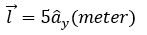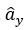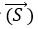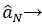# Basics of electromagnetic field theory

In this section we will discuss about the basic definitions and formulas of electromagnetic field theory which will be helpful for you. This section is very much important for you to understand this subject.

• x,y,z represents 3 distance variable ( 3 dimensions).
• POINT:
1. a point is a sphere of radius r tends to zero (r→0)
2.  Also we can say that point is a meet of three surfaces ( like a corner of a  room where three surfaces meet).
3. A point has no variables ( as it has zero radius) but it has three constants.

Ex:       P (1,2,3) be a point where x=1, y=2, z=3

All three (x,y,z) are constants

• LINE:
1. when we add or join many points in a particular direction then it will create a line . so a line has magnitude and direction both so it is a vector.

2. Line can be considered as a cylinder of radius tends to zero(r→0).

3. As I told you above that line has a direction, it means it has a variable. And this also means that it has two constants.

Ex.Here, 5 is the magnitude andis the unit vector in the direction of y.

• SURFACE
1. By adding or joining no. o lines in series we can get a surface and hence it has two dimensions or two directions or two variables or one constant.

2. It has a direction hence it is a vector quantity.

• Surface vectoris defined as

Where ,Normal unit vector to the surface in outward direction.

The formula above is generated because the surface is a vector and a vector has direction so we have to give the direction. Now to give the direction of a surface we choose the axis which is perpendicular(normal) to it as shown in figure below.

In the fig. above the normal to the surface will give the position of that surface that is why we have five given the normal vector.

If you want to buy led bulbs, then this will be the best deal. Check out this.

For above example,

• VOLUME
1. We consider volume as a one whole unit like in above example the whole cuboid is considered as a volume, if we put something in that then it will be considered to be putted in that cuboid . Hence we the volume is no more a vector , it is a scalar.
2. A volume has 3 variables and zero constants.

we will discuss some more concepts and definitions in further posts. thank you.